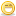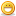# Thread: Tutorial: Binary, hex and decimal .. oh my!

1. ##Tutorial: Binary, hex and decimal .. oh my!

After a little discussion with some users in IRC, I was asked on how I teach the conversation from hex to binary to decimal. So I've decided to post everything here along the lines that I usually teach in class.

First, let's look at binary. It is merely a 1 (on) or 0 (off). We see binary used in a variety ways from permissions (in unix) to tcp/ip addresses to attribute settings. (space out the bit so that a decimal appears under each 0)

0 0 0 0 0 0 0 0
128 64 32 16 8 4 2 1

So if my binary was like below:

0 1 1 0 1 1 0 0
128 64 32 16 8 4 2 1

This means that the bits 64, 32, 8, 4 are turned on totalling a value of 108 (64+32+8+4).

If you have to go beyond the 8 bits (the examples above are 8 bits as found in IPv4 addressing aka 32bit addressing --> 4x8bits), just continue doubling the numbers. So if I had to go to 12 bits (aka Fat12) I would have:

0 0 0 0 0 0 0 0 0 0 0 0
2048 1024 512 256 128 64 32 16 8 4 2 1

And so on.

Simple, eh? Now, how about Hex? Well Hex aka Hexadecimal is a "base-16" numbering system. Binary is a "base-2" (based on 2 digits) and decimal is "base-10" (based on 10 digits).

If I was to count in binary and decimal it would be:

Binary = Decimal
0000 = 0
0001 = 1
0010 = 2
0011 = 3
0100 = 4
0101 = 5
0110 = 6
0111 = 7
1000 = 8
1001 = 9
1010 = 10
1011 = 11
1100 = 12
1101 = 13
1110 = 14
1111 = 15

So, now I can count easily from 0 to 15. Oh. Interesting. 16 digits.We'll add the hex to the digit now.

0000 = 0 = 0
0001 = 1 = 1
0010 = 2 = 2
0011 = 3 = 3
0100 = 4 = 4
0101 = 5 = 5
0110 = 6 = 6
0111 = 7 = 7
1000 = 8 = 8
1001 = 9 = 9
1010 = 10 = A
1011 = 11 = B
1100 = 12 = C
1101 = 13 = D
1110 = 14 = E
1111 = 15 = F

So now I can go from binary to decimal to Hex. This little chart is easy to setup and handy to have. Once you have this the rest is straightforward. An example:

0 1 1 0 1 0 1 0 1 1 1 0
2048 1024 512 256 128 64 32 16 8 4 2 1

Notice that there are 12 bits. Seperate your bits into groups of 4 like so:

0 1 1 0 1 0 1 0 1 1 1 0

And do the hex for each (hex is easier than decimal). This example would come out to:

6 A E

Sometimes written as 6AExh. Now do the decimal:

0 1 1 0 1 0 1 0 1 1 1 0
2048 1024 512 256 128 64 32 16 8 4 2 1

So, 1024+512+128+32+8+4+2 = 1,710(barring any addition errors that should be it). Most of my students get errors in the decimal portion due to addition errors. Give it a try and its best to do it a few times on paper to practise. Once you get that down, it will be straightforward after that.

If you want some more examples, let me know and I will put some more up.

Hope this helps.Reply With Quote

2. ## i hope you...

i hope you write tutorials...thanx for the mini-lesson... although i knew this already, i encourage you to make tutorials and such and perhaps start your own website? cuz then, you know, you could shamelessly promote your website here too, like adore from hackers doesReply With Quote

3. ##Thank you. As I come up with more things that are needed and that I have some clue about I will post accordingly.Is there something that you'd like to see a tutorial about?Reply With Quote

4. ##not any specifics... i just was trying to encourage you. its nice to see people who take the initiative to share information.Reply With Quote

5. how a bout a quick and down and dirty java tutorial? i have the class now and i'm learning the why's but its a little slow on the what to do if you wanna... stuff.. does that make sense ?Reply With Quote

6. ##Java, unfortunately, ain't my speciality. But if you ask in the Security Programming forum perhaps someone there might be able to help.

I tend to be more network admin oriented. I'm working on my programming these days.Reply With QuoteReply With Quote

8. ##Ok.. I will work on that one over the weekend and see what I can come up.Subnetting, the lost art and also it's evil twin, supernetting.Reply With Quote

9. ##great job...

hey greate job mittens....i learned a lot...good job...
and thanks for the tutorial ...

hey mittens..if u know assembly why don't u write something on it and post....i am sure that will help a lot..

thanks once again..

intruder...Reply With Quote

10. ##If your up to it..

How 'bout mathematical operations in hex and binary? addition, subtraction, multiplication, division, etc. I work on it in my free time, but school takes up most of it and anionline and learning programming the rest.

Thanks for the excellent binary/decimal/hex basics, too.Reply With Quote

####Posting Permissions

• You may not post new threads
• You may not post replies
• You may not post attachments
• You may not edit your posts
•